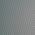Sunday, June 12, 2016

When does n divide a_n in this sequence?

Consider the following sequence:

a(1)=0

a(2)=2

a(3)=3

for all n ≥ 4  a(n) = a(n-2)+a(n-3)

Here is a table of a(n) for 2 ≤ n ≤ 23

n       2     3     4      5      6     7       8      9    10     11     12
a(n)  2     3     2      5     5      7     10    12    17     22     29

n       13    14    15    16       17      18     19      20     21      22      23
a(n) 39    51    68    90    119   158   209   277   367   486   644

For 2≤ n ≤ 23 the n such that n divides a(n) are

n = 2,3,5,7,11,13,17,19,23.

Notice a pattern? Of course you do!

I propose the following question which I will answer in my next post (in a week or so)

PROVE or DISPROVE the following:

for all n≥ 2   n divides a(n) iff n is prime.

1.I spent some time trying to prove this, but it turns out to be false! One can check that 271441 divides a(271441), but 271441 = 521^2 is not prime.

1.You are correct- 561^2 is the first counterexample.
I am surprised you found it so fast!

2.I wrote a program to test it out on the first half a million values and also found the counter-example at 271,441 (the program ran out of heap space before hitting 500,000 so I don't know where the next counter-example is yet). So, is there anything interesting or special about that number?

3.The second counterexample is 904631.

2.For one direction of your proof, I hope you make use of the fact that these numbers count maximal independent sets in cycles.

3.counter example: 134 divides a(134)
a(134)/134 = 23149346804971524/134 = 172756319440086

1.Something not quite exact here.

23149346804971526 / 134 does yield a quotient of 172756319440086, but with a remainder of 2.

4.Used a python script to conclude that 271441 is indeed a counter-example.[Did not read the comment above!] But we need to see if primality is sufficient for the divisibility.

5.Interesting that the first counterexample is a perfect square. It suggests possibly weakening the conjecture: Maybe every n that divides a(n) is either a prime or a prime power?

Alas no. The second counterexample is 904631 = 7 x 13 x 9941.

6.So, like the run of nine 8s in Pi, pointing out the difference between noticing a pattern over a finite range and proving that pattern is true on an infinite range.

7.I had a simpler "proof" that the conjecture is false: if it were true, it would give a very past primality testing algorithm and GASARCH would publish this algorithm somewhere other than this blog. This sequence is similar to a Fibonacci sequence and so (see Matrix Form in the wikipedia article on Fibonacci numbers) one can compute a(n) in time log(n)*(time required to multiply two numbers of size a(n)). One can compute a(n) mod n in time log(n)*(time required to do arithmetic mod(n)), so one can compute it in time O(log(n)^3).

8.Proof by OEIS: http://oeis.org/A013998

9.One observation I managed to make is this: a(n) is divisible by n if n is a prime or pseudoprime. Both 271,441 and 904,631 are Perrin's pseudoprimes.

1.Aren't Perrin's pseudoprimes defined by this exact problem?

10.can you prove the following sentence plz?

"If n is a prime, n divides A(n)"